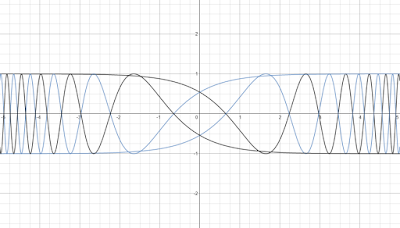## Friday, August 21, 2015

### Fun with Desmos

Some graphs with the Desmos free online graphing calculator, which can be found at https://www.desmos.com/.  Desmos is also available as an iOS app and Android app.

Cosine Graphs in a Bottle
y= cos(2^x), -cos(2^x), cos(2^-x), -cos(2^-x)y= cos(2^x), -cos(2^x), cos(2^-x), -cos(2^-x)

red curve: x^3+y^4 = 6 sin(12*x^-2)
blue curve: -x^3+y^4 = 6 sin(12*x^-2)red: x^3+y^4 = 6 sin(12*x^-2), blue: -x^3+y^4 = 6 sin(12*x^-2)
A Spirograph
polar curve: r = 6 sin (2 sin (θ - 2 (cos (θ^1.02)))polar curve: r = 6 sin (2 sin (θ - 2 (cos (θ^1.02)))

y^2 = (8.65 * exp(x))/(-3*x^0.2 -3*x^2 + x^4)y^2 = (8.65 * exp(x))/(-3*x^0.2 -3*x^2 + x^4)

Double Teardrop Squeezed on One Side
right: x = 4.5(-cos t + 1), y = 4 * sin t * sin (0.5*t^2)
left:  x = 3 * (cos t - 1), y = sin t * (0.5*t)^4
-4 π ≤ t ≤ 4 πright: x = 4.5(-cos t + 1), y = 4 * sin t * sin (0.5*t^2)left:  x = 3 * (cos t - 1), y = sin t * (0.5*t)^4
Vibration
1.025*x*y^2 = 1.5*π*sin(x^3)1.025*x*y^2 = 1.5*π*sin(x^3)
x = 0.65*t + 1.5 sin(t^2)
y = 5 * (sin t)^3
-4 π ≤ t ≤ 4 πx = 0.65*t + 1.5 sin(t^2) y = 5 * (sin t)^3

Get Desmos and have some fun!

Eddie

This blog is property of Edward Shore.  2015

### Texas Instruments: TI-36X Pro and TI-30X Pro Mathprint

Essentially, the Texas Instruments TI-36X Pro and the TI-30X MathPrint are functionally equivalent.  What makes the two calculators differe...